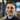Blog

# TLA+ verifying a money transfer

December 07, 2019

What could go wrong with transferring money between two accounts? I have written about how easy it is to get wrong (giving away or losing money) and patterns for preventing problems like with the outbox pattern. TLA+ provides a language and tooling for proving systems that might be able to help us understand if we have design flaws. The first example from TLA+ is about transferring money from alice to bob, this is the basic premise:

Alice and bob have accounts We want to transfer money from alice to bob No account can go negative Invariant: total money in the system should be constant at all times

In the example they end with an incomplete example:

---- MODULE Transfer ----
EXTENDS Naturals, TLC

(* --algorithm transfer
variables alice_account = 10, bob_account = 10,
account_total = alice_account + bob_account;

process Transfer \in 1..2
variable money \in 1..20;
begin
Transfer:
if alice_account >= money then
MoveMoney: alice_account := alice_account - money;
bob_account := bob_account + money;
end if;
C: assert alice_account >= 0;
end process

end algorithm *)

\* BEGIN TRANSLATION
\* ...
\* END TRANSLATION

MoneyInvariant == alice_account + bob_account = account_total

Where we have a race condition that violates our money invariant. Can you spot it? Critically, the MoveMoney step happens after the balance check on alice’s account, both processes will pass the balance check in parallel and then potentially bring the account into negative. A solution would be to keep the balance check and money movement in the same step to prevent the race:

\* ...
Transfer:
if alice_account >= money then
alice_account := alice_account - money;
bob_account := bob_account + money;
end if;
\* ...

Now lets say these balances are on different systems (microservices maybe?) that are unable to atomicly operate on each other, what would a locking mechanism look like? To change the setup, we will need to update each money movement line to its own steps: MoveAlice, MoveBob - to describe them no longer being atomic.

Now how to counteract the loss of atomicity? We can start with awaiting on a locked flag that only one process at a time can hold. Running this and having it fail might come as a little surprise, since the lock prevents concurrency of processes for balance changes, but that is not where our invariant is being violated.

(what was the invariant again?)

Since the balances are on different steps, the invariant MoneyInvariant == alice_account + bob_account = account_total is impossible to enforce. Our system guarantees this is true eventually after an individual process has completed, but during that processes execution we have no atomic operator to guarantee that in this setup.

What needs to change for the system to validate? We have to relax our invariant MoneyInvariant == alice_account + bob_account <= account_total, and then inside our process update the logic to always withdraw then deposit, which we can assert has been balanced on processes completion. This is similar to the money being ‘in transit’ and unavailable until the processes have settled.

---- MODULE Transfer ----
EXTENDS Naturals, TLC

(* --algorithm transfer
variables alice_account = 10, bob_account = 10, locked = 0,
account_total = alice_account + bob_account;

process Tranfer \in 1..2
variable money \in 1..20

begin
Transfer:
await locked = 0;
locked := 1;
Move:
if alice_account >= money then
MoveAlice: alice_account := alice_account - money;
MoveBob: bob_account := bob_account + money;
end if;
Finish:
locked := 0;
assert alice_account + bob_account = account_total;

end process
end algorithm *)

\* BEGIN TRANSLATION
\* ...
\* END TRANSLATION

MoneyEventualInvariant == alice_account + bob_account <= account_total

Now if you are interested, you could add an invariant to represent the eventual consistency. When no processes are running so at some point we can say MoneyInvariant == alice_account + bob_account = account_total. We mentioned microservices earlier, if these were different services we would also need to add in the possibility that a process would crash halfway through - where one account would have money withdrawn and not yet deposited in the second.Written by Andrew Klotz. Building tools to help people to do their best. You should follow him on Twitter